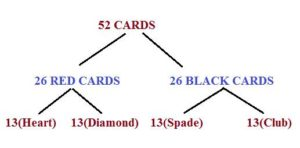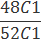# PROBLEMS ON PROBABILITY:PART 2-HOW TO SERIES

Hi Bankersdaily Aspirants,

Learning is a step by step process and you should gradually progress in that to ace the process. As you all know we have started a new How to Series to help with your preparations for various exams like IBPS PO , IBPS RRB Scale I officer , IBPS RRB Office Assistant , IBPS Clerk , IBPS SO , SBI PO , SBI Clerk , SBI SO and many other exams in this type.

Friends as I always say to ace in the exam we don’t want to get Cent Percentage, we just want to get the cut off mark in the exam.It is not that much difficult to get the cut off mark.There is certain topic in the Aptitude Section which we can solve in less than a minute & PROBABILITY is one among that.If you have practiced this topic then you can solve this with in a minute.We had already discussed some of the types of questions  in Previous Article(Probability Part 1).Today we will discuss another set of question in this article.Before that have a glance of Part 1

TOPICS TO BE DISCUSSED

1)BASED ON CARDS

2)BASED ON  BALLS

## #.1.TYPE 1:

### BASED ON CARDS:

Friends in the last Article we had discussed problems on cards with the Keyword Both.In this Article we will discuss problems based on the Keyword

No, One from one type & Another from another type### 13 Cards in Diamond,Heart,Spade & Club Contains:

Numbered Card:2,3,4,5,6,7,8,9,10(Totally 9  Numbered Cards)

Face Card:There are Face Card they are (ACE,KING QUEEN & JACK)

9+4=13 CARDS in each Suit.

Now let us look into the Problem.

1)When 1 card is drawn at Random,What is the Probability that there will be NO King Card?

EXPLANATION

Probability=Number of Possible Ways/Total Number of Events

Here we are going to draw 1 card therefore the Total number of Events or Sample Space=52C1=52

There are 4 King Cards in 52 Cards

1 from Diamond,1 from Heart,1 from club,1 from Spade,Totally 4 King Cards  in 52 Cards.

=52-4=48

Therefore Possible ways=48C1

Probability of getting No King:=48/52

=12/13

## Friends shall we solve together,

3 Cards are drawn,Total Possibility=52C3=52*51*50/1*2*3

=26*17*50

Now,let us find the Possible Number of ways of getting 1 Ace,1 King & 1 Jack

There are 4 Ace in 52 Cards,

1 King in 52 Cards,

1 Jack in 52 Cards,

Now,we have to choose 1 card from each face.

4C1 * 4C1 * 4C1=4*4*4

Therefore,

=4*4*4/ 26*17*50

Probability of getting 3 Cards=16/5525

## #.2.TYPE 2:

### BASED ON  BALLS:

In this type,Questions will be asked in such a way that certain balls are drawn in different colours and after that there will be two conditions after this

1)They were Replaced.

2)They were not Replaced.

1)THEY WERE REPLACED:

A bag contains 8 Red & 10 Black Balls.If two draws of three balls are drawn randomly & the balls are replaced after the first draw then what is the  probability that three  balls are Red Balls at the first draw and  Black Balls at the Second Draw?

EXPLANATION

A Bag Contains 8 Red & 10 Black Balls

At First Draw:

They will draw three balls of Red Colour,

Number of Possible ways=8C3(Since 3 Red Balls are to be drawn from 8 Red Balls)

Total Number of Ways=18C3(Since 3 Red Balls are to be drawn from 18 Balls)

We all know the formula to find the Probability,

=Number of Possible Ways/Total Number of Ways

=8C3/18C3

8C3=8*7*6/1*2*3=56

18C3=18*17*16/1*2*3=816

=56/816

Probability of the First Draw=7/102

At Second Draw:

We want to draw 3 Balls from 10 Black Balls

Possible Ways=10C3=10*9*8/1*2*3=120

Total Number of Ways=18C3=18*17*16/1*2*3=816

Probability=120/816=5/34

Probability of the Second Draw:5/34

### Total Probability=7/102 * 5/34

We had Used * here Do You know why?

Because In the Question they ask us to find the Probability of  First Draw & Second Draw

And is Used So we have to Multiply it,

If they said to Find First Draw or Second Draw then We would add it .

Note:If “AND” is represented in the question then we have to “Multiply” the term.

If “OR” is represented in the question then we have to “Add” the term.

### 2)Without Replacement:

A Bag Contains 8 Red Balls & 10 Black Balls.Four Balls are Drawn one by one & they are not replaced.Find the Probability if the balls drawn are of Alternate of different Colours?(That is,if 1st ball is Red 2nd would be Black …,Vice Versa)

EXPLANATION

Let us Assume if 1st Ball Drawn of Red Colour then,

Probability of 1st Ball:

Possible Ways=8C1=8

Total Ways=18C1=18

Probability of First Ball=8/18=4/9

Since the Ball is not Replaced,now there will be 7Red Balls & Black Balls Remain the Same Totally 17 Balls

Probability of 2nd Ball(That is Black):

Possible Ways=10C1=10

Total Ways=17C1=17

Probability of Second Ball=10/17

This Ball will also be not Replaced then there will be 7Red Balls 9 Black Balls & the Total number of Balls=16 balls

Probability of Third Ball:(Red)

Possible Ways=7C1=7

Total Ways=16C1=16

Probability of Third Ball=7/16

This Ball also will not be replaced then there will be 6 Red balls & 9 Black Balls & the total number of Balls=15 Balls

Probability of Fourth Ball(Black)

Possible Ways=9C1=9

Total Ways=15C1=15

Probability of Fourth Ball=9/15=3/5

Since we want all the Four ball(AND) Therefore we must Multiply individual Probability

4/9 * 10/17 * 7/16 * 2/5

Probability of drawing 4 balls alternatively( if we start with Red Ball)=7/102

First Ball(Black)=10/18=5/9

Second Ball(Red)=8/17

Third Ball(Black)=9/16

Fourth Ball(Red)=7/15

When Four Balls are drawn the Probability is=7/102

Now the Probability is First Ball May be RED or Second Ball May be BLACK

Now we have to add because the Term OR is used

7/102 + 7/102 =14/102=7/51